# Samacheer Kalvi 12th Maths Solutions Chapter 2 Complex Numbers Ex 2.2

## Tamilnadu Samacheer Kalvi 12th Maths Solutions Chapter 2 Complex Numbers Ex 2.2

Question 1.
Evaluate the following if z = 5 – 2i and w = -1 + 3i
(i) z + w
(ii) z – iw
(iii) 2z + 3w
(iv) zw
(v) z2 + 2zw+ w2
(vi) (z + w)2
Solution:
(i) z = 5 – 2i, w = -1 + 3i
z + w = (5 – 2i) + (-1 + 3i)
= (5 – 1) + (-2i + 3i)
= 4 + i
(ii) z – iw = (5 – 2i) – i (-1 + 3i)
= 5 – 2i + i + 3
= (5 + 3) + (-2i + i)
= 8 – i
(iii) 2z + 3w = 2(5 – 2i) + 3 (-1 +3i)
= 10 – 4i – 3 + 9i
= 7 + 5 i
(iv) zw = (5 – 2i) (-1 + 3i)
= -5 + 15i + 2i – 6i2
= -5 + 17i + 6
= 1 + 17i
(v) z2 + 2zw + w2 = (z + w)2 [from (i)]
= (4 + i)2
= 16 – 1 + 8i
= 15 + 8i
(vi) (z + w)2 = 15 + 8z [from (v)]Question 2.
Given the complex number z = 2 + 3i, represent the complex numbers in Argand diagram.
(i) z, iz, and z + iz
(ii) z, -iz, and z – iz
Solution:
(i) z = 2 + 3i
iz = i(2 + 3i)
= (2i – 3)
= -3 + 2i
z + iz = (2 + 3i) + (-3 + 2i)
= -1 + 5i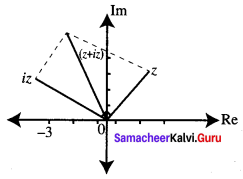(ii) z = 2 + 3i
-iz = -i(2 + 3i)
= -2i – 3i2
= (3 – 2i)
z – iz = (2 + 3i) + (3 – 2i)
= 5 + i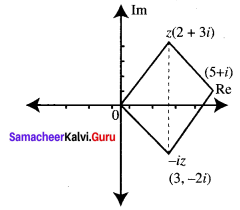Question 3.
Find the values of the real numbers x and y, if the complex numbers.
(3 – i) x – (2 – i) y + 2i + 5 and 2x + (-1 + 2i) y + 3 + 2i are equal
Solution:
(3 – i) x – (2 – i) y + 2i + 5 = 2x + (-1 + 2i) y + 3 + 2i
⇒ 3x – ix – 2y + iy + 2i + 5 = 2x – y + 2yi + 3 + 2i
⇒ (3x – 2y + 5) + 1 (-x + y + 2) = (2x – y + 3) + i (2y + 2)
Equate real parts on both sides
3x – 2y + 5 = 2x – y + 3
x – y = -2 ……. (1)
Equate imaginary parts on both sides
-x + y + 2 = 2y + 2
-x – y = 0
x + y = 0 ……. (2)
(1) + (2) ⇒ 2x = -2
x = -1
Substituting x = -1 in (2)
-1 + y = 0
⇒ y = 1
∴ x = -1, y = 1### Samacheer Kalvi 12th Maths Solutions Chapter 2 Complex Numbers Ex 2.2 Additional Problems

Question 1.
Find the real values of x and y, if
(i) (3x – 7) + 2 iy = -5y + (5 + x)i
(ii) (1 – i)x + (1 + i)y = 1 – 3i
(iii) (x + iy)(2 – 3i) = 4 + i
(iv)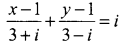Solution:
(i) We have (3x – 7) + 2 iy = 5y + (5 + x)i
⇒ 3x – 7 = 5y and 2y = 5 + x
⇒ 3x + 5y = 7 and x – 2y = -5
⇒ x = -1 y = 2

(ii) We have, (1 – i) x + (1 + i)y = 1 – 3i
⇒ (x + y) + i(-x + y) = 1 – 3i
⇒ x + y = 1
and -x + y = 3 [On equating real and imaginary parts]
⇒ x = 2 and y = -1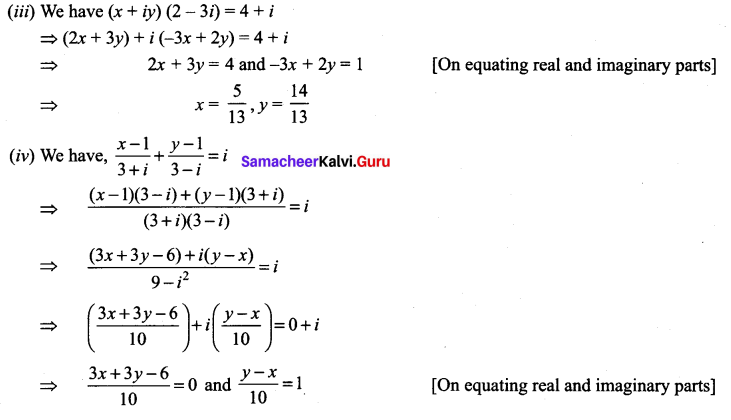⇒ x + y – 2 = 0 and y – x = 10
⇒ x = -4 , y = 6.Question 2.
Find the real values of x and y for which the complex numbers -3 + ix2y and x2 + y + 4i are conjugate of each other.
Solution:
Since -3 + ix2y and x2 + y + 4i are complex conjugates.
∴ -3 + ix2y = x2 + y + 4i
… (0 and, x2 y = -4 …. (ii)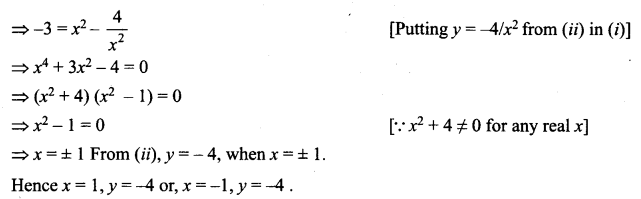Question 3.
Given x = 2 – 3i and y = 4 + i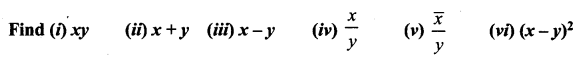Solution:
Do it yourself